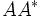# Unitary group is conjugacy-closed in general linear group

This article gives the statement, and proof, of a particular subgroup in a group being conjugacy-closed: in other words, any two elements of the subgroup that are conjugate in the whole group, are also conjugate in the subgroup
View a complete list of such instances/statements

## Statement

Let$GL(n,\mathbb{C})$ denote the general linear group: the group of invertible$n \times n$ complex matrices. Let$U(n,\mathbb{C})$ denote the unitary group: the subgroup comprising matrices$A$ such that$AA^*$ is the identity matrix. Then,$U(n,\mathbb{C})$ is conjugacy-closed in$GL(n,\mathbb{C})$: any two unitary matrices that are conjugate over$GL(n,\mathbb{C})$, are conjugate in$U(n,\mathbb{C})$.

## Proof

The proof uses the following facts:

• By the spectral theorem for unitary matrices, any unitary matrix is conjugate, in the unitary group, to a diagonal unitary matrix.
• Also, clearly any two diagonal unitary matrices that are conjugate in the general linear group, are conjugate by a permutation matrix, hence they are conjugate in the unitary group.

Thus, we have established a conjugate-dense subgroup of$U(n,\mathbb{C})$ (namely the diagonal unitary matrices) such that any two elements of the subgroup are conjugate in$GL(n,\mathbb{C})$ iff they are conjugate in$U(n,\mathbb{C})$. This shows that$U(n,\mathbb{C})$ is conjugacy-closed in [itex]GL(n,\mathbb{C}).